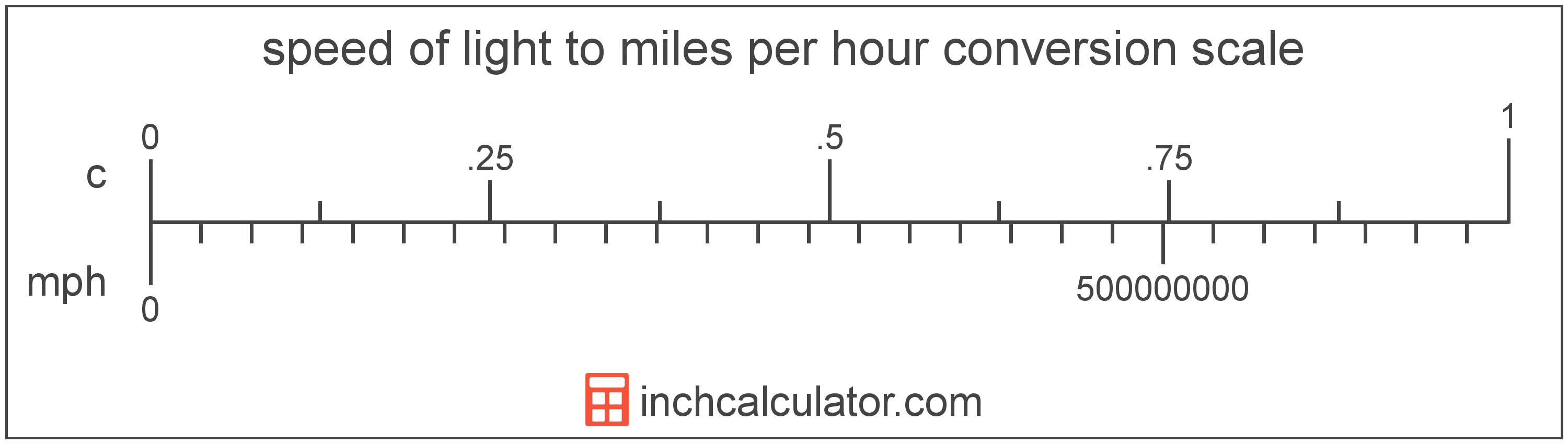# Miles per Hour to Speed of Light Converter

Enter the speed in miles per hour below to get the value converted to speed of light.

Results in Speed of Light:1 mph = 1.4912E-9 c
Hint: use a scientific notation calculator to convert E notation to decimal

Do you want to convert speed of light to miles per hour?

## How to Convert Miles per Hour to Speed of Light

To convert a measurement in miles per hour to a measurement in speed of light, multiply the speed by the following conversion ratio: 1.4912E-9 speed of light/mile per hour.

Since one mile per hour is equal to 1.4912E-9 speed of light, you can use this simple formula to convert:

speed of light = miles per hour × 1.4912E-9

The speed in speed of light is equal to the speed in miles per hour multiplied by 1.4912E-9.

For example, here's how to convert 500,000,000 miles per hour to speed of light using the formula above.
speed of light = (500,000,000 mph × 1.4912E-9) = 0.745582 cMiles per hour and speed of light are both units used to measure speed. Keep reading to learn more about each unit of measure.

## What Are Miles per Hour?

Miles per hour are a measurement of speed expressing the distance traveled in miles in one hour.

The mile per hour is a US customary and imperial unit of speed. Miles per hour can be abbreviated as mph, and are also sometimes abbreviated as mi/h or MPH. For example, 1 mile per hour can be written as 1 mph, 1 mi/h, or 1 MPH.

Miles per hour can be expressed using the formula:
vmph = dmi / thr

The velocity in miles per hour is equal to the distance in miles divided by time in hours.

## What Is the Speed of Light?

The speed of light is equal to exactly 299,792,458 meters per second, or 670,616,629 miles per hour. The definition of the speed of light is actually derived from the most recent 1983 international definition of the meter.

Speed of light can be abbreviated as c; for example, 1 speed of light can be written as 1 c.

## Mile per Hour to Speed of Light Conversion Table

Table showing various mile per hour measurements converted to speed of light.
Miles Per Hour Speed Of Light
1 mph 0.0000000014912 c
2 mph 0.0000000029823 c
3 mph 0.0000000044735 c
4 mph 0.0000000059647 c
5 mph 0.0000000074558 c
6 mph 0.000000008947 c
7 mph 0.000000010438 c
8 mph 0.000000011929 c
9 mph 0.00000001342 c
10 mph 0.000000014912 c
100 mph 0.00000014912 c
1,000 mph 0.0000014912 c
10,000 mph 0.000014912 c
100,000 mph 0.000149 c
1,000,000 mph 0.001491 c
10,000,000 mph 0.014912 c
100,000,000 mph 0.149116 c
1,000,000,000 mph 1.4912 c

## References

1. Wikipedia, Miles per hour, https://en.wikipedia.org/wiki/Miles_per_hour
2. National Institute of Standards and Technology, SI Redefinition: Meter, https://www.nist.gov/si-redefinition/meter